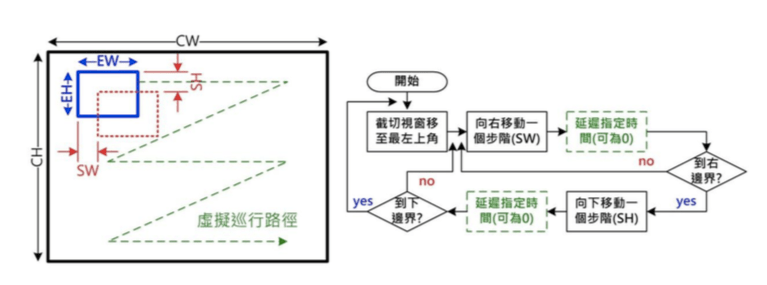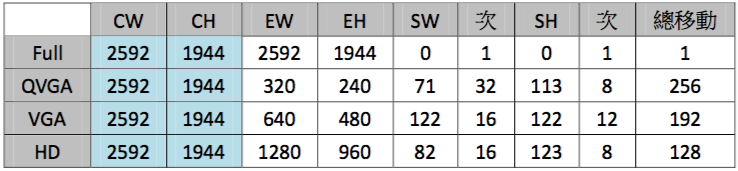# 【Tutorial】OpenQCam 在ePTZ攝影機的應用T=((CW–EW)/SW)*((CH-EH)/SH)*ST （公式一）

CW: 取像水平解析度， CH: 取像垂直解析度。

EW: 影像截切水平解析度， EH: 影像截切垂直解析度。

SW: 水平移動步階， SH: 垂直移動步階。

ST: 為步階移動間隔時間，可自由指定，通常希望以 1 個影格(Frame)時間 1/30 秒為間隔，但實際上會因 Pi Zero 處理速度來不及可能須降至 1/15~1/5 秒。T = ((CW – EW) / SW) * ((CH-EH) / SH) * ST
= ((2592 – 640) / 122) * ((1994 – 480) / 122) * 60ms = 16 * 12 * 60ms = 11.520 sec

Mat matCap;// 儲存原始影像

cap >> matCap;// 從攝像頭取得影像並存到 matCap 中

//取得原始影像 matSrc 中指定位置及大小(Rect(左上 X 座標，左上 Y 座標，寬度， 高度))的影像到 matROI
Mat matROI = matSrc(Rect(begin_x, begin_y, width, height));

// 宣告 LCD 顯示及原始影像縮小圖用矩陣

Mat matDisp;

Mat matCapResize;

// 將取得之 ROI 影像縮小至 320*240 畫像素並放到 matDisp 中
// 一般縮放採雙線性內差(CV_INTER_LINEAR)就夠用，如果不在乎影像品質將縮 放速度加快一點，可採用 CV_INTER_NEAREST 內插法。
cv::Resize(matROI, matDisp, cv::Size(320, 240), 0, 0, CV_INTER_LINEAR);

// 將原始影像縮小至 80*60 畫像素後貼至顯示區左上角

Mat matDispROI = matDisp(Rect(0, 0, 80, 60));

cv::Resize(matCap, matCapResize, cv::Size(80,60), 0, 0, CV_INTER_NEAREST);

matCapResize.CopyTo(matDispROI);

### 延伸閱讀 — 完整程式

main.cpp

#include <stdio.h> #include <iostream> #include “ILI9341.h” #include <opencv2/opencv.hpp> #include <ctime>

#define MAX_W 2592

#define MAX_H 1944

using namespace std; using namespace cv;

int main(int argc, char **argv) {

// 巡行參數 0:原始解析度 / 1:QVGA / 2:VGA / 3:HD int para_num = 0; // 巡行參數編號 int EW = {2592, 320, 640, 1280}; // 裁切影像寬度

// 垂直移動距離 int EH = {1944, 240, 480, 960}; int SW = {2592, 71,122,82}; int SH = {1994, 113, 122, 123}; int curr_x = 0; // 目前巡行起點 X int curr_y = 0; // 目前巡行起點 Y int curr_ew = EW; // 目前裁切影像寬度 int curr_eh = EH; // 目前裁切影像高度 int curr_sx = SW; // 目前水平移動距離 int curr_sh = SH; // 目前垂直移動距離 // 裁切影像高度 // 水平移動距離 double t0,t1,t2; int result = LCD_Inital(); // LCD 初始化

switch(result){ // 顯示初始化錯誤訊息 case 0:

printf(“LCD inital OK.\n”);

break; case 1:

printf(“BCM2835 inital failed. Are you running as root??\n”);

break; case 2:

printf(“GPIO inital failed. Are you running as root??\n”);

break; case 3:

printf(“SPI inital failed. Are you running as root??\n”);

break; case 4:

printf(“ILI9341 inital failed. Please check PCB connection is OK.\n”);

break; default:

printf(“LCD inital failed.\n”);

}

if(result != 0) return 0;

VideoCapture cap(0); // 啟動攝像頭連續取像

if (!cap.isOpened()) { // 若無法開啟則結束 cerr << “ERROR: Unable to open the camera” << endl; return 0;

}

cap.set(CV_CAP_PROP_FRAME_WIDTH, 2592); // 設定攝像頭輸入為最大解析度 cap.set(CV_CAP_PROP_FRAME_HEIGHT,1944);

Mat matCap; Mat matCapResize; Mat matROI; Mat matDisp; int count = 0;

cout << “Start grabbing !” << endl; LCD_SetHorizontalDisplay(); // 設定 LCD 為橫式顯示

char strFps; // 儲存速度字串 t1 = (double)getTickCount(); // 取得目前時間

while(1) { for(curr_y=0; curr_y<MAX_H-EH[para_num]; curr_y += SH[para_num]){

for(curr_x=0; curr_x<MAX_W-EW[para_num]; curr_x += SW[para_num]){ t0 = t1; // 儲存舊時間 t1 = (double)getTickCount(); // 儲存目前時間

cap >> matCap; // 將取得的影像複製到 matCap

if(para_num == 0){ resize(matCap, matDisp, cv::Size(320,240), 0, 0, INTER_LINEAR); // 將影

else{ matROI = matCap(Rect(curr_x,curr_y,curr_ew,curr_eh)); // 裁切指定位

Mat matDispROI = matDisp(Rect(0, 0, 120, 90)); // 指定 ROI 區

rectangle(matCap, cv::Point(curr_x, curr_y), cv::Point(curr_x+EW[para_num],curr_y+EH[para_num]),

Scalar(0,0,255), 20); // 在原影像上畫 ROI 框 resize(matCap, matCapResize, cv::Size(120,90), 0, 0, INTER_NEAREST); //

// 計算兩次執行時間差 取倒數即為每秒幀數 顯示在 LCD 左上角 // 若不需顯示則註解掉下面兩行 sprintf(strFps,”%2.1f FPS”, 1.0 / ((t1-t0) / getTickFrequency())); putText(matDisp, strFps, Point(200,20), FONT_HERSHEY_DUPLEX, 0.8,

Scalar(0,255,0),1);

// 將影像逐行顯示在 LCD 上 for(int i = 0; i < matDisp.rows; i++){

char *ptrS = matDisp.ptr<char>(i);

ILI9341_WriteLineBGR2RGB565(ptrS, matDisp.cols); }

// 若無法取得影像則結束程式 if (matCap.empty()) {

cerr << “ERROR: Unable to grab from the camera” << endl;

return 0;

}

count ++;

if(count%2 == 0) // 工作中 LED1 閃爍 bcm2835_gpio_write(PIN_GPIO_LED_R, 1); // 點亮 LED1

else bcm2835_gpio_write(PIN_GPIO_LED_R, 0); // 熄減 LED1

// 若 SW1 按下則切換到下一組巡行參數 if(bcm2835_gpio_lev(PIN_GPIO_SW1) != 0){

delay(10); // 去除按鍵彈跳 curr_x = MAX_W; // 強迫離開雙 for loop curr_y = MAX_H; //

}

// 若 SW2 按下則綠燈閃一下結束程式 if(bcm2835_gpio_lev(PIN_GPIO_SW2) != 0){

bcm2835_gpio_write(PIN_GPIO_LED_G, 1); // 點亮 LED2 delay(50); bcm2835_gpio_write(PIN_GPIO_LED_G, 0); // 熄減 LED2 bcm2835_gpio_write(PIN_GPIO_LED_R, 0); // 熄減 LED1 cap.release();

BCM2835_End(); cout << “Done!” <<endl; return 0;

}

if(curr_x >= MAX_W) break;

} // end of for loop curr_x

if(curr_y >= MAX_H) break;

} // end of for loop curr_y para_num ++;

if(para_num >= 4) para_num = 0;

curr_ew = EW[para_num]; // 目前裁切影像寬度 curr_eh = EH[para_num]; // 目前裁切影像高度 curr_sx = SW[para_num]; // 目前水平移動距離 curr_sh = SH[para_num]; // 目前垂直移動距離

} // end of while

}

sudo ./go.sh (編譯程式)
sudo ./ePTZ (執行程式)

（本文同步發表於歐尼克斯實境互動工作室（OmniXRI）文章連結；責任編輯：葉于甄。）Author: 許 哲豪